## General Question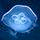# 10 parts per billion ozone (MW=48) equals how much ozone in ug/m3?

Asked by sshonkoff (28) July 9th, 2009

how do I figure this out?

Observing members: 0Composing members: 0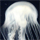Tink (8673)“Great Answer” (0) Flag as…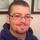Huh?

loser (15027)“Great Answer” (0) Flag as…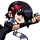Those units are not directly compatible. You would have to know something specific about the density of ozone in a particular area.

Ivan (13479)“Great Answer” (1) Flag as…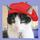I’ll get back to you after I figure out your question. (Don’t hold your breath.)

gailcalled (54631)“Great Answer” (0) Flag as…It has been a long time since I studied chemistry. Here is my strategy, but check out my assumptions to make sure they are right. I think a mole is the number of molecules of air in 22.4 m^3 at STP. I suppose it would be sufficient to work with just 79% nitrogen and 21% oxygen, or whatever the percentages are. Divide the molecular weights by 22.4 and multiply by the corresponding percentages. Then the weight of the ozone in this m^3 of air would be 10/billion times the total weight. I don’t think you need to work with the molecular weight of ozone.

LostInParadise (29755)“Great Answer” (0) Flag as…Oh, and multiply by 1000 to convert liters to m^3

LostInParadise (29755)“Great Answer” (0) Flag as…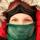Wow. An ozone question.

So you are given a mixing ratio (you have 10 ozone molecules out of a total billion “other” molecules). I convert this not so useful unit to number density all the time, which isn’t exactly what you want, but I think the conversion from number density to density is probably pretty straight forward.

First, I’m going to assume you’re working at standard temp and pressure. We’ll use SI units but I’ll let you do the conversions since this does have an awful homework vibe to it, so your standard temp 0 Celsius and your standard pressure is 1 atmosphere (kind of. Some people use 0.986 atm for standard pressure, but lets be easy). Now, the first thing you have to do is convert your mixing ratio into a partial pressure (the pressure the ozone would have if it, alone, occupied the volume of the total gas). The formula for mixing ratio is given as : (Partial pressure)/(ambient pressure). Solve for Partial pressure (you know the other two).

Great. Now you should have a number (less than 1) with a unit of Pascals. If you don’t check your work.

Now we can solve for number density using the Ideal Gas Law (one of my personal favorites): Pressure = K*(rho)*T where Pressure is now your partial pressure, K is the Boltzman constant, rho is the density and T is temperature (STP). You can look up K (check your units!), but solve this for density (rho). You should get a number with units (# per m^3).

Now, here’s where I stop (this is good for my work), but you’ll need to convert this to g per m^3. I’m pretty sure this can be as simple as finding out what the molecular weight of ozone is (I know, but I’m going to have you look it up) and converting your number density using that.

Hope this helps.

BTW, Not that it may make much of a difference, but I’m an ozone scientist, so I do these things a lot.

Les (9640)“Great Answer” (0) Flag as…or